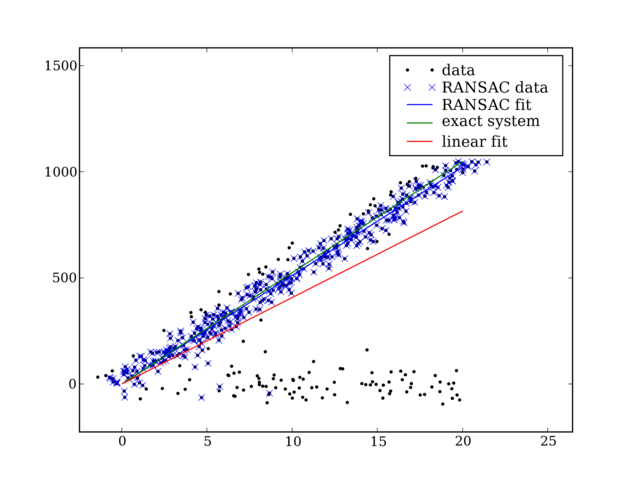# RANSAC¶

The attached file ransac.py implements the RANSAC algorithm. An example image:To run the file, save it to your computer, start IPython

In [ ]:
ipython -wthread


Import the module and run the test program

In [ ]:
import ransac
ransac.test()


To use the module you need to create a model class with two methods

In [ ]:
def fit(self, data):
"""Given the data fit the data with your model and return the model (a vector)"""
def get_error(self, data, model):
"""Given a set of data and a model, what is the error of using this model to estimate the data """


An example of such model is the class LinearLeastSquaresModel as seen the file source (below)

ransac.py

Section author: AndrewStraw, DatChu

Attachments